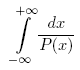ENG  RUS Timus Online JudgeOnline Judge
Problems
Authors
Online contests
Site news
Webboard
Problem set
Submit solution
Judge status
Guide
Register
Authors ranklist
Current contest
Scheduled contests
Past contests
Rules

## 1621. Definite Integral

Time limit: 1.0 second
Memory limit: 64 MBwhere P(x) = a4 · x4 + a3 · x3 + a2 · x2 + a1 · x + a0. P(x) has no real roots and GCD(P(x), P'(x)) = const.

### Input

The input contains five integers: a0, a1, a2, a3 and a4 separated by whitespace. Each of these numbers does not exceed 106 by absolute value, a4 ≠ 0.

### Output

Output the value of the integral. Assume that the exact value is A and your answer is B. Your answer will be considered correct if and only if at least one of the following statements is true:
• A − 10−9BA + 10−9
• A · (1 − 10−9) ≤ BA · (1 + 10−9)

### Sample

inputoutput
`16 0 0 0 1`
```0.2776801836
```
Problem Source: SPbSU ITMO contest. Petrozavodsk training camp. Winter 2008.